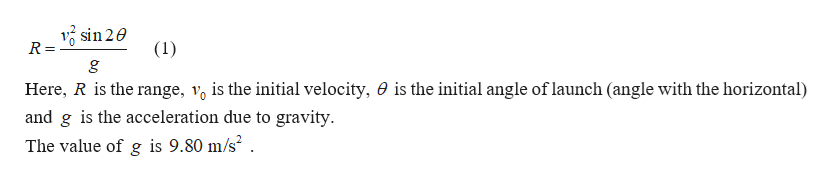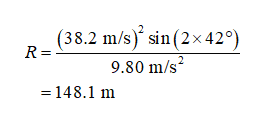A golf ball is given an initial velocity of 38.2 m/s at an angle of 42.0o above the horizontal. How far away will the ball land on level ground?

Question

A golf ball is given an initial velocity of 38.2 m/s at an angle of 42.0o above the horizontal. How far away will the ball land on level ground?

Step 1

The golf ball will be in a projectile motion. The maximum horizontal distance reached by the ball is the range of the ball. The equation for the range of the projectile motion is given byhelp_outlineImage Transcriptionclosesin 2e R = (1) g Here, R is the range, vo is the initial velocity, 0 is the initial angle of launch (angle with the horizontal) and g is the acceleration due to gravity. The value of g is 9.80 m/s2 . fullscreen
Step 2

Substitute the numerical val...help_outlineImage Transcriptionclose(38.2 m/s) sin (2x 42°) R = 9.80 m/s2 = 148.1 m fullscreen

Want to see the full answer?

See Solution

Want to see this answer and more?

Our solutions are written by experts, many with advanced degrees, and available 24/7

See Solution
Tagged in

Physics